ROOT   Reference Guidedouble32.C File Reference

## Detailed DescriptionTutorial illustrating use and precision of the Double32_t data type You should run this tutorial with ACLIC: a dictionary will be automatically created.

root > .x double32.C+

The following cases are supported for streaming a Double32_t type depending on the range declaration in the comment field of the data member:

Case Declaration
A Double32_t fNormal;
B Double32_t fTemperature; //[0,100]
C Double32_t fCharge; //[-1,1,2]
D Double32_t fVertex; //[-30,30,10]
E Double32_t fChi2; //[0,0,6]
F Int_t fNsp;
Double32_t* fPointValue; //[fNsp][0,3]
• Case A fNormal is converted from a Double_t to a Float_t
• Case B fTemperature is converted to a 32 bit unsigned integer
• Case C fCharge is converted to a 2 bits unsigned integer
• Case D the array elements of fVertex are converted to an unsigned 10 bits integer
• Case E fChi2 is converted to a Float_t with truncated precision at 6 bits
• Case F the fNsp elements of array fPointvalue are converted to an unsigned 32 bit integer. Note that the range specifier must follow the dimension specifier.

Case B has more precision than case A: 9 to 10 significative digits and 6 to 7 digits respectively. The range specifier has the general format: [xmin,xmax] or [xmin,xmax,nbits]. Examples

• [0,1]
• [-10,100];
• [-pi,pi], [-pi/2,pi/4],[-2pi,2*pi]
• [-10,100,16]
• [0,0,8] Note that:
• If nbits is not specified, or nbits <2 or nbits>32 it is set to 32
• If (xmin==0 and xmax==0 and nbits <=14) the double word will be converted to a float and its mantissa truncated to nbits significative bits.

## IMPORTANT NOTE

Lets assume an original variable double x. When using the format 0,0,8 you get the best relative precision when storing and reading back the truncated x, say xt. The variance of (x-xt)/x will be better than when specifying a range for the same number of bits. However the precision relative to the range (x-xt)/(xmax-xmin) will be worse, and vice-versa. The format [0,0,8] is also interesting when the range of x is infinite or unknown.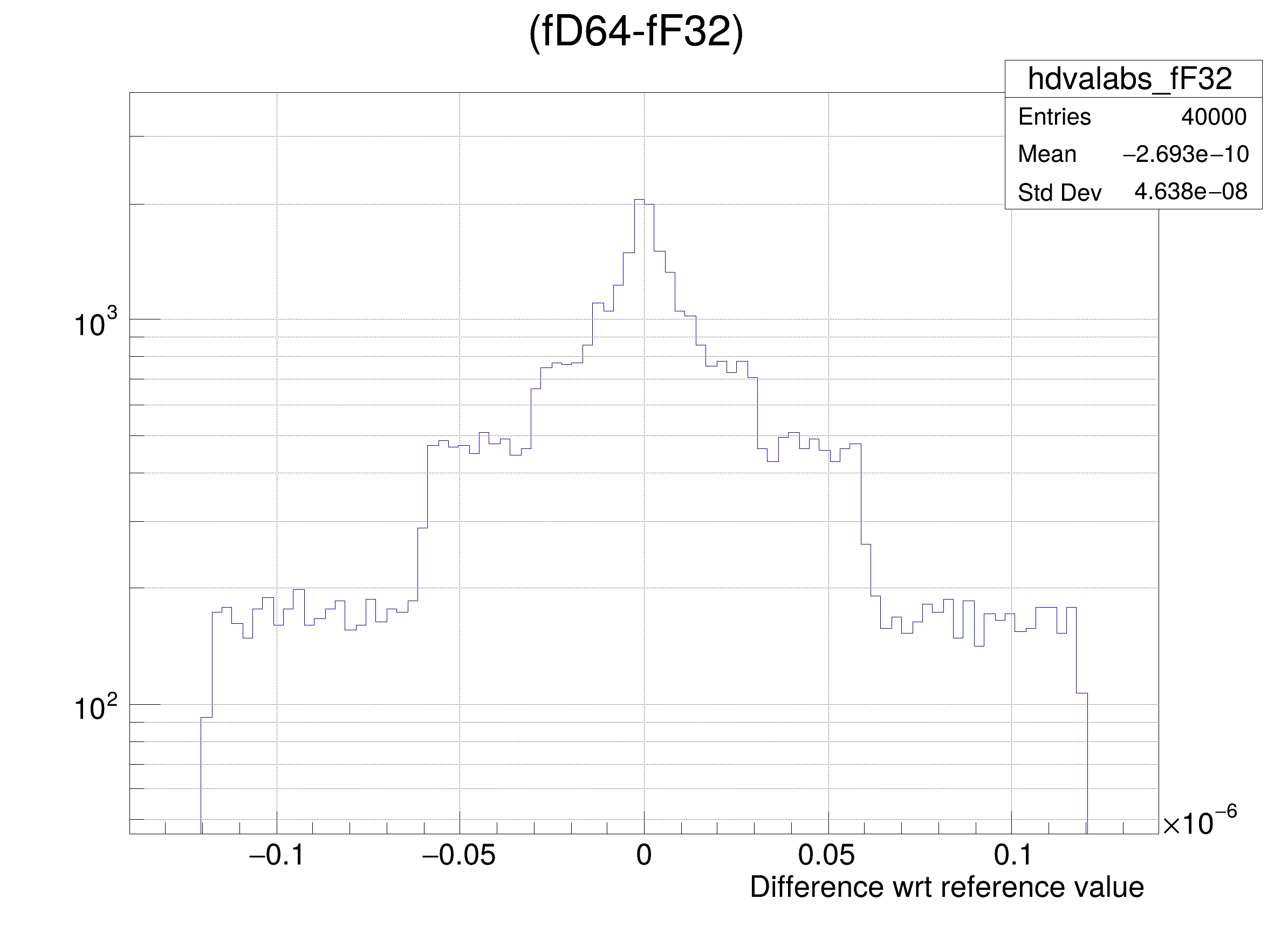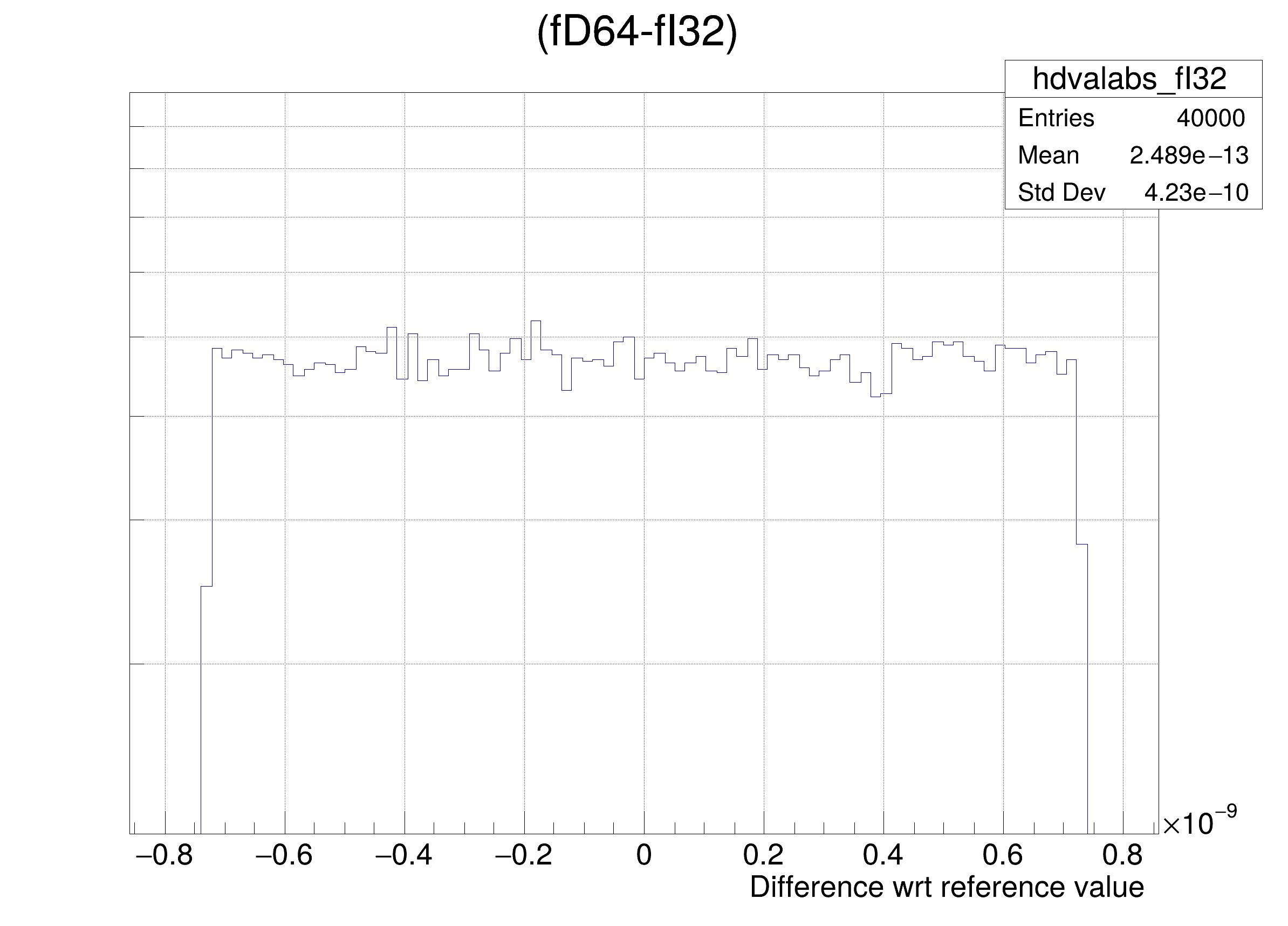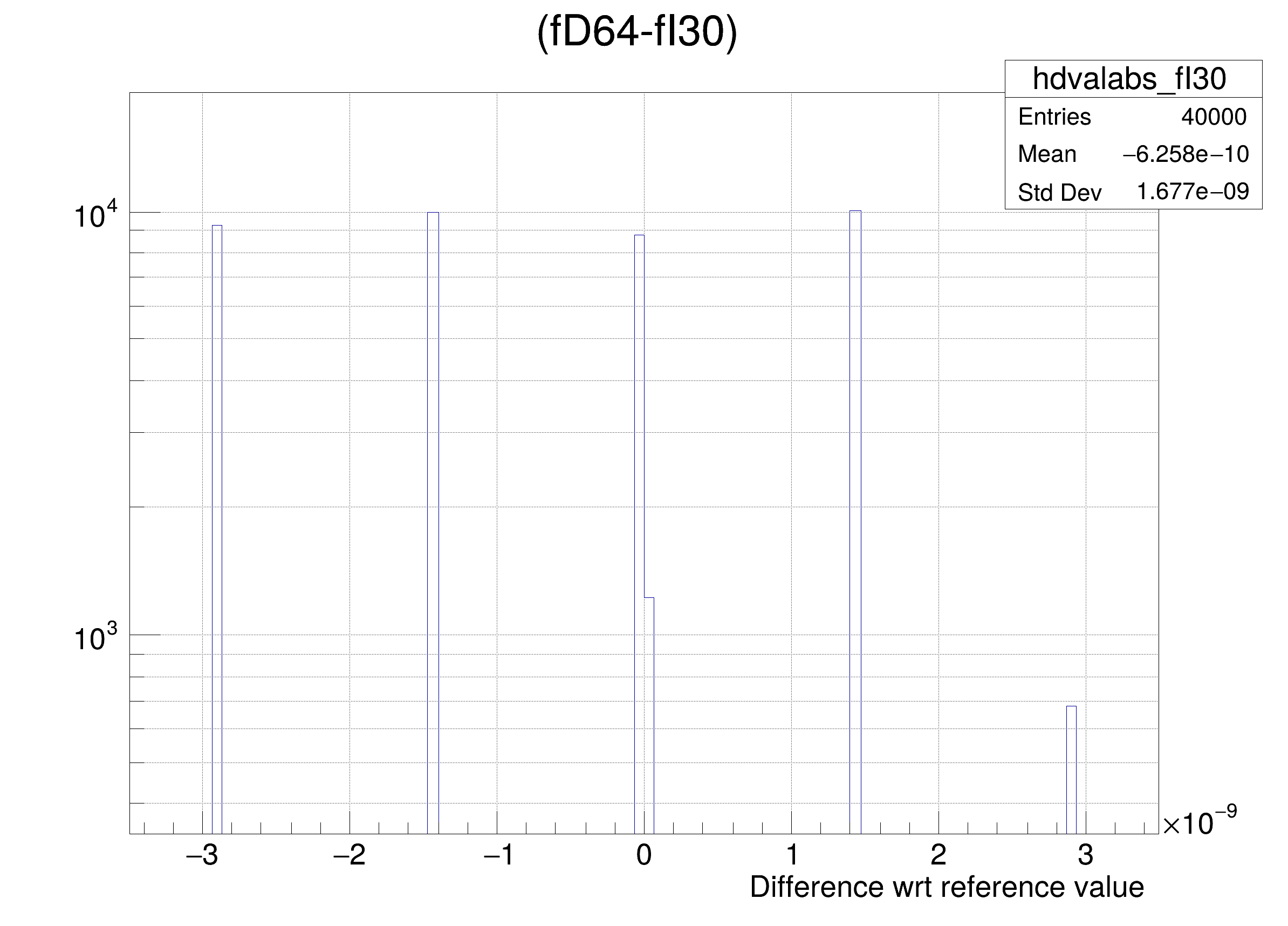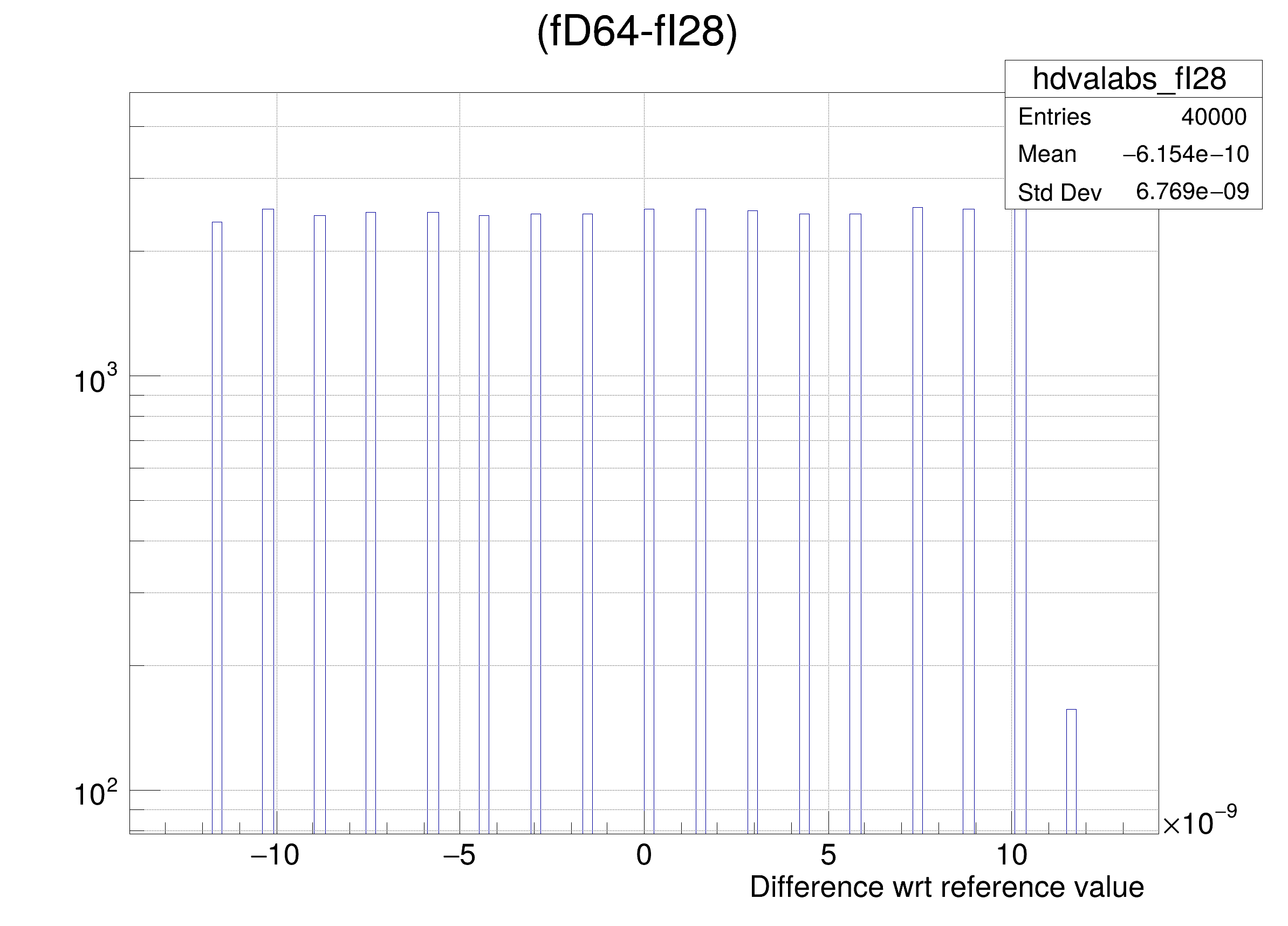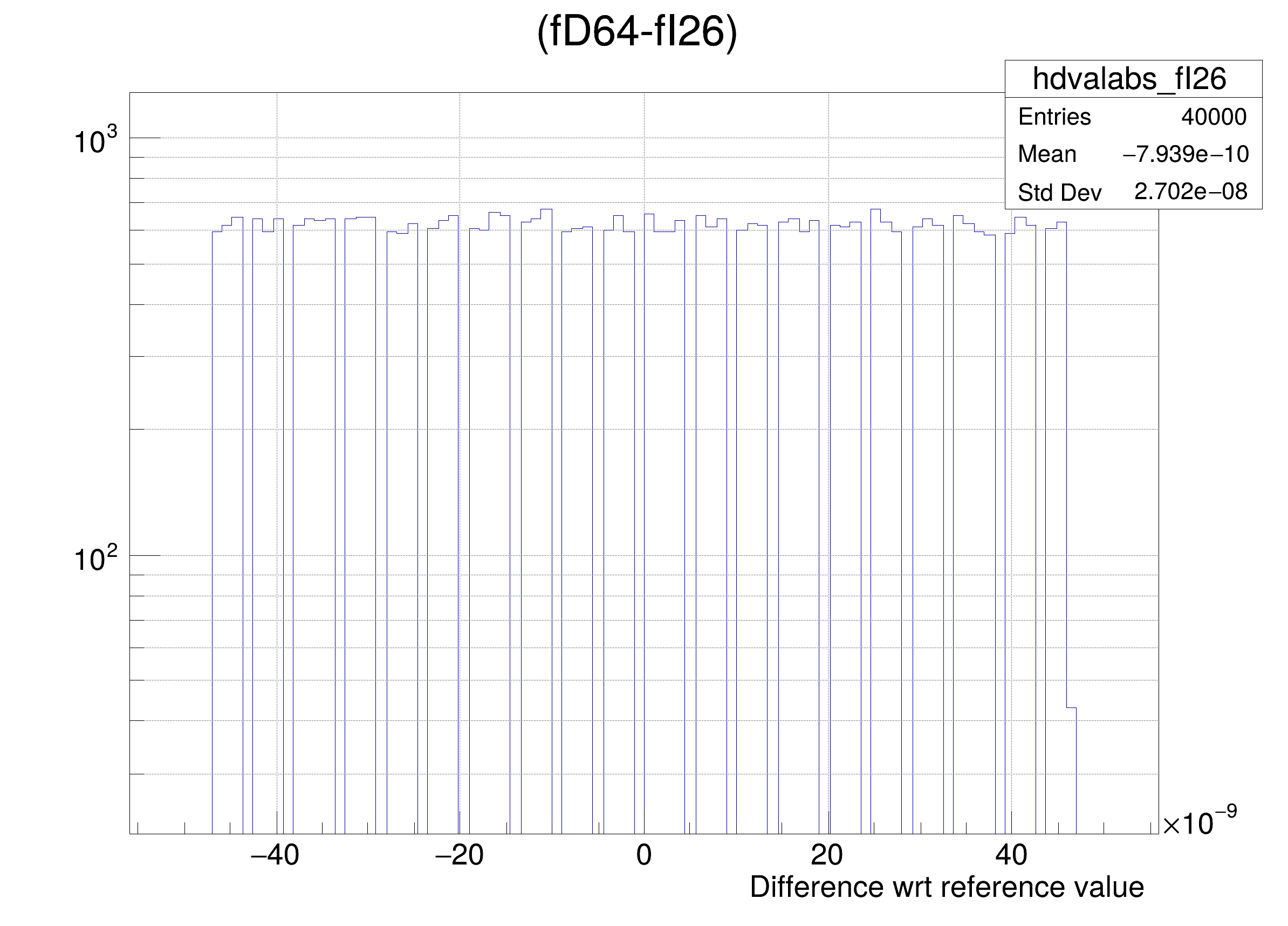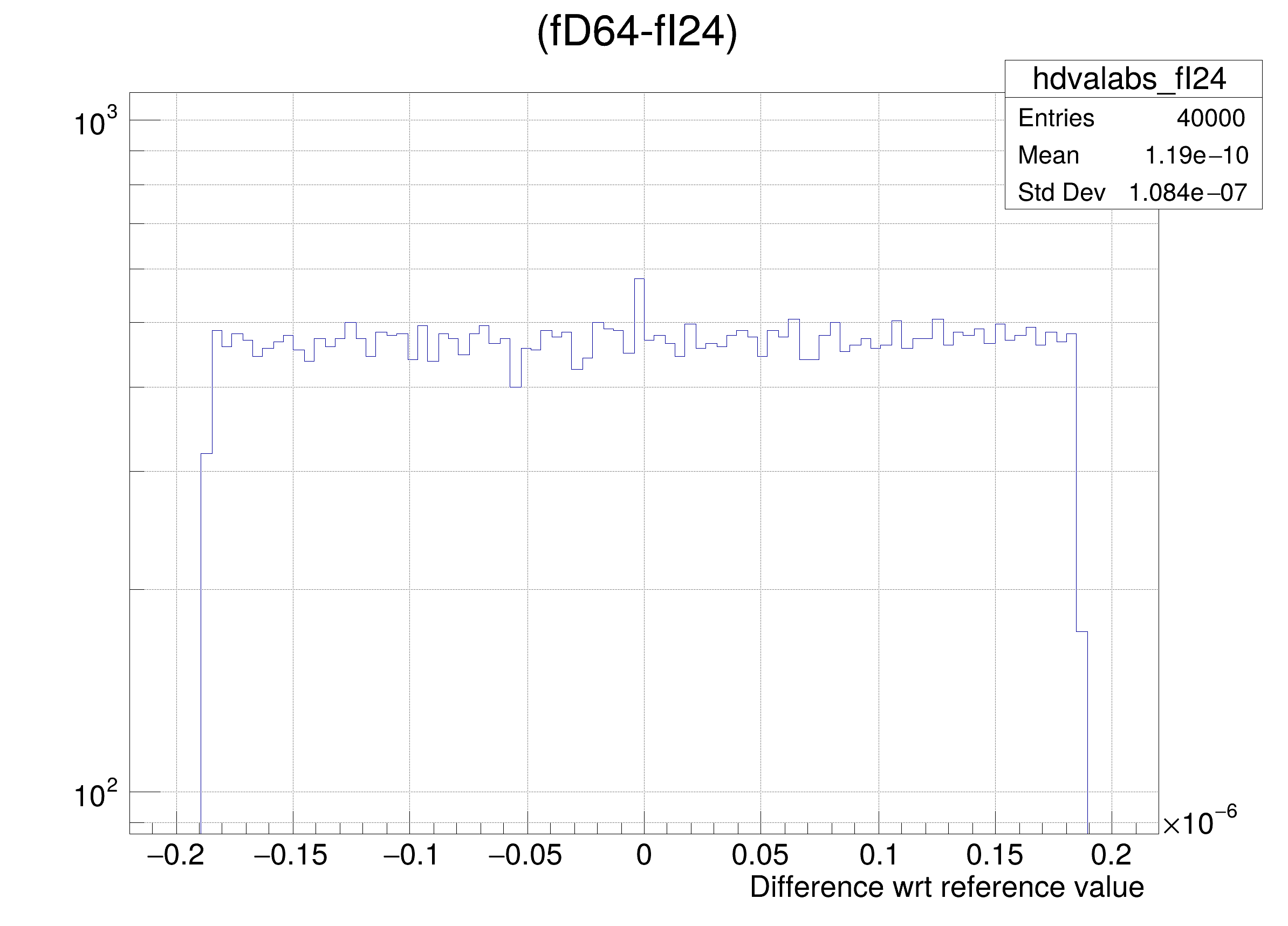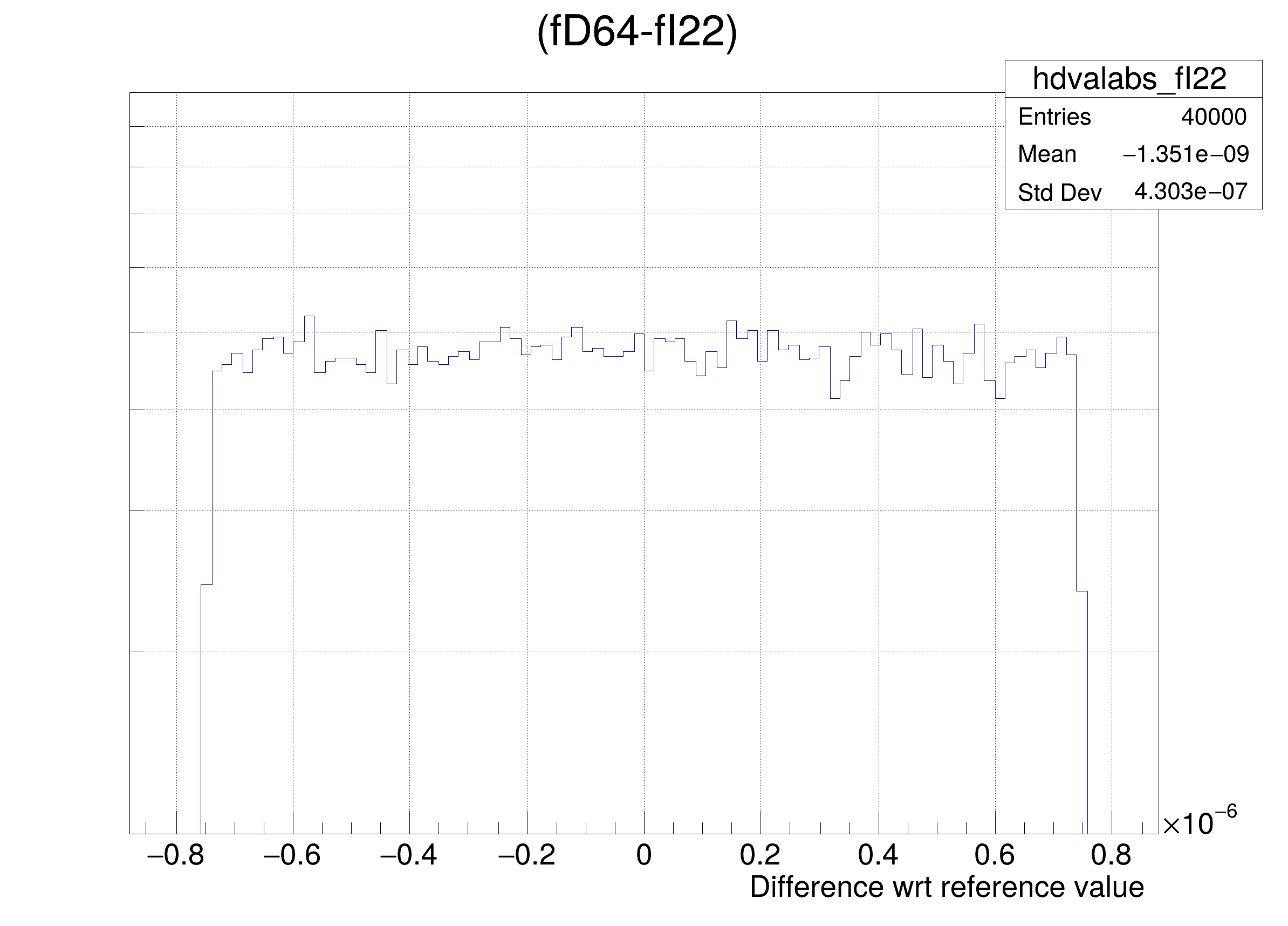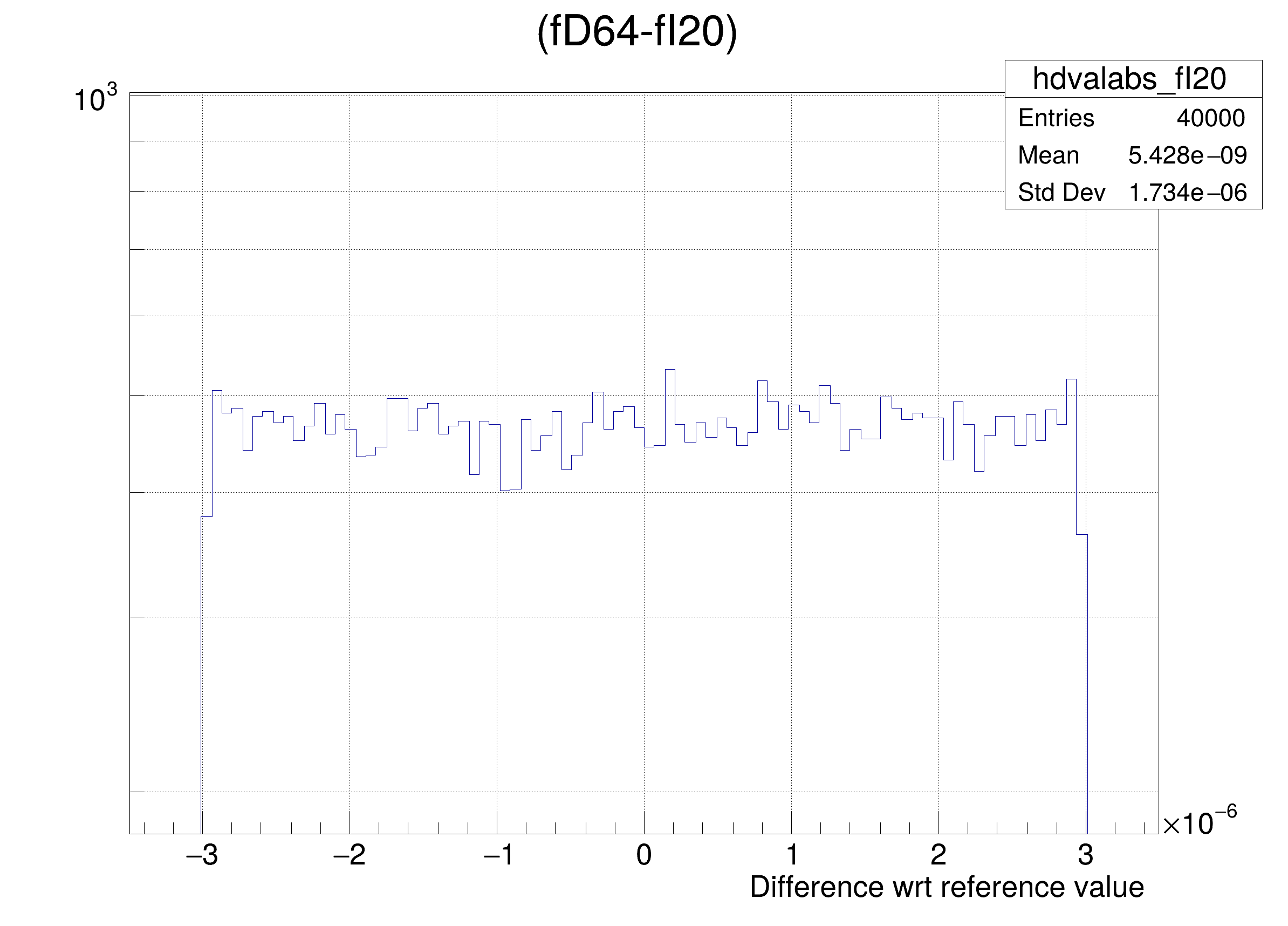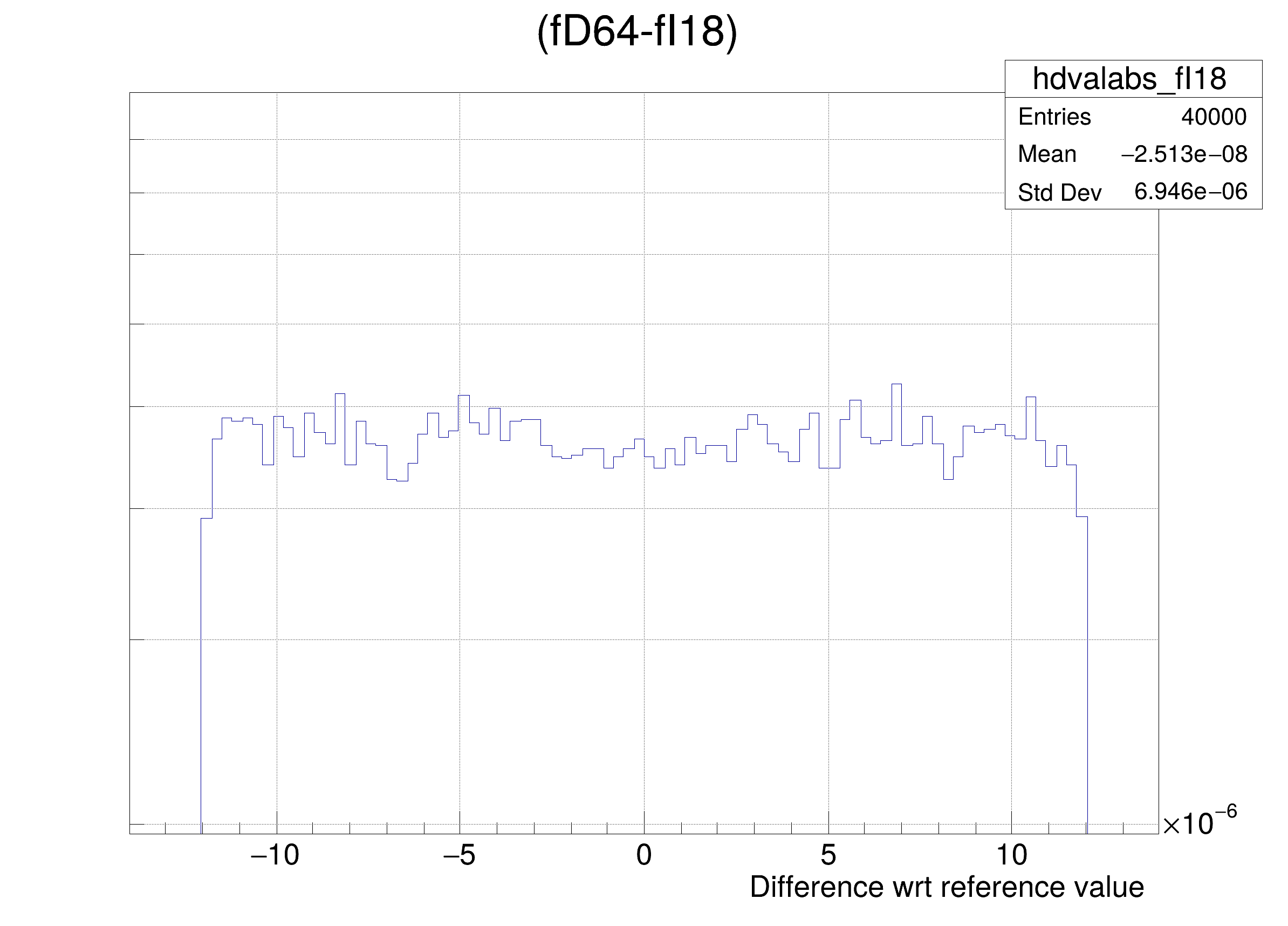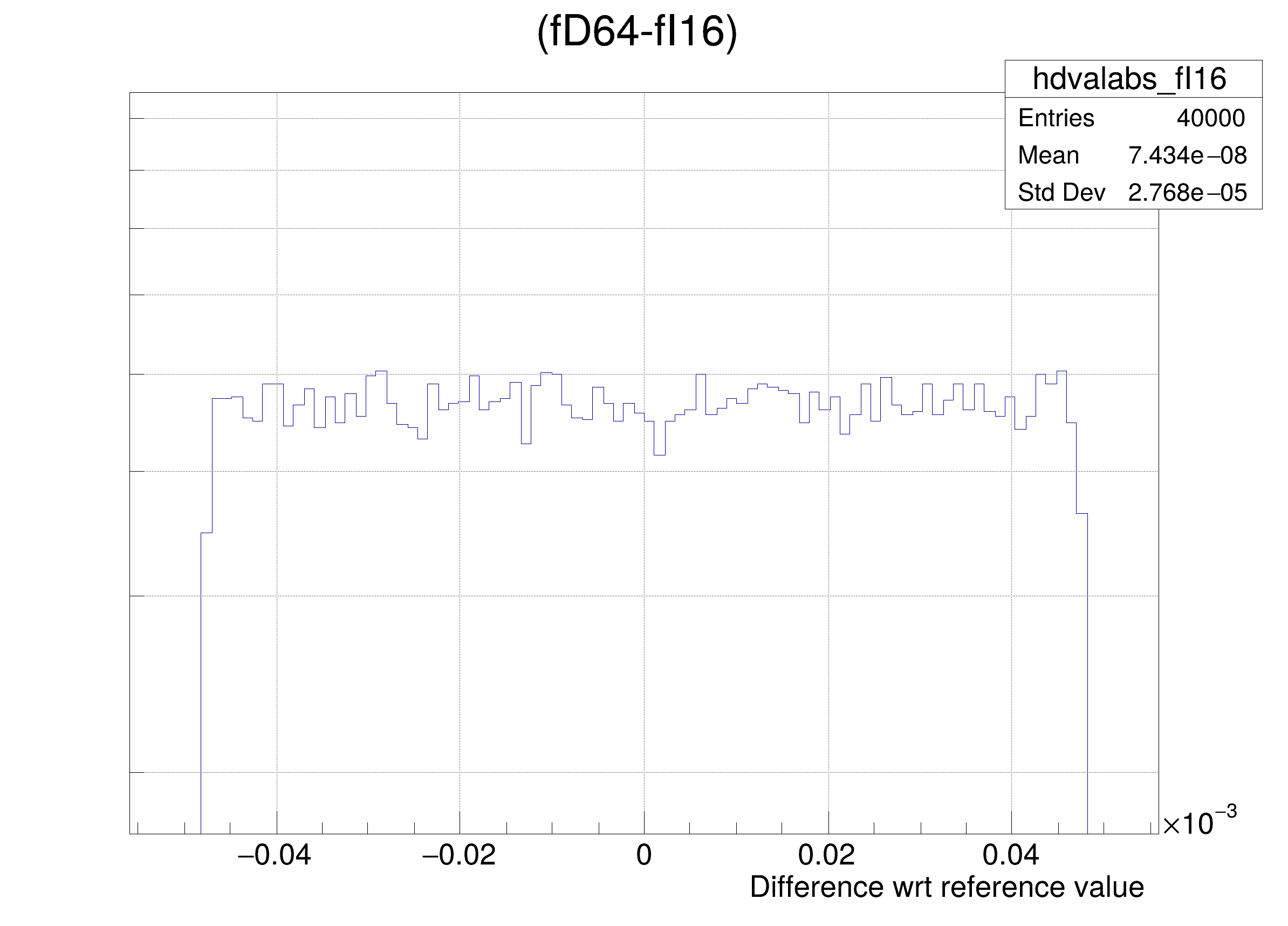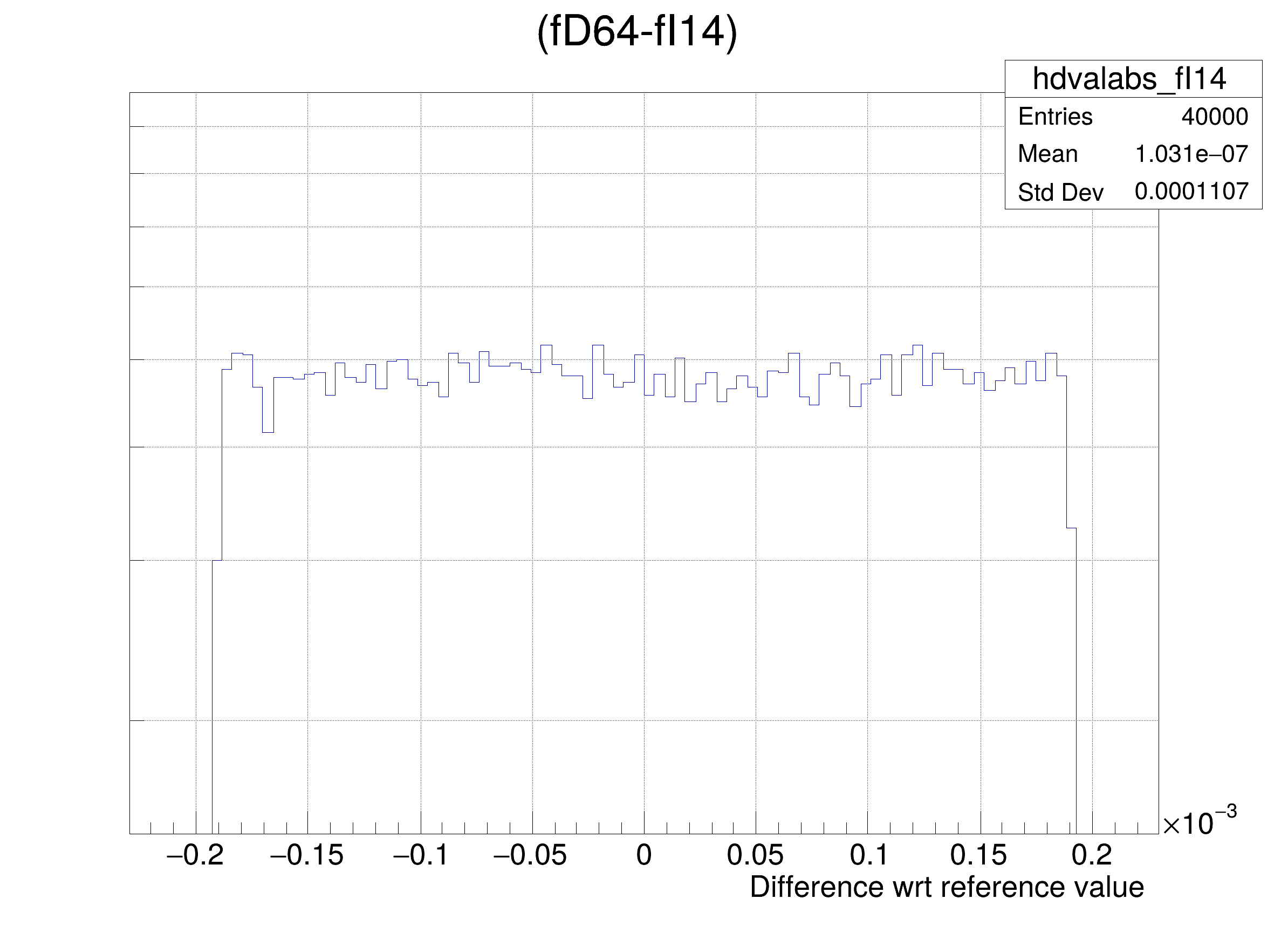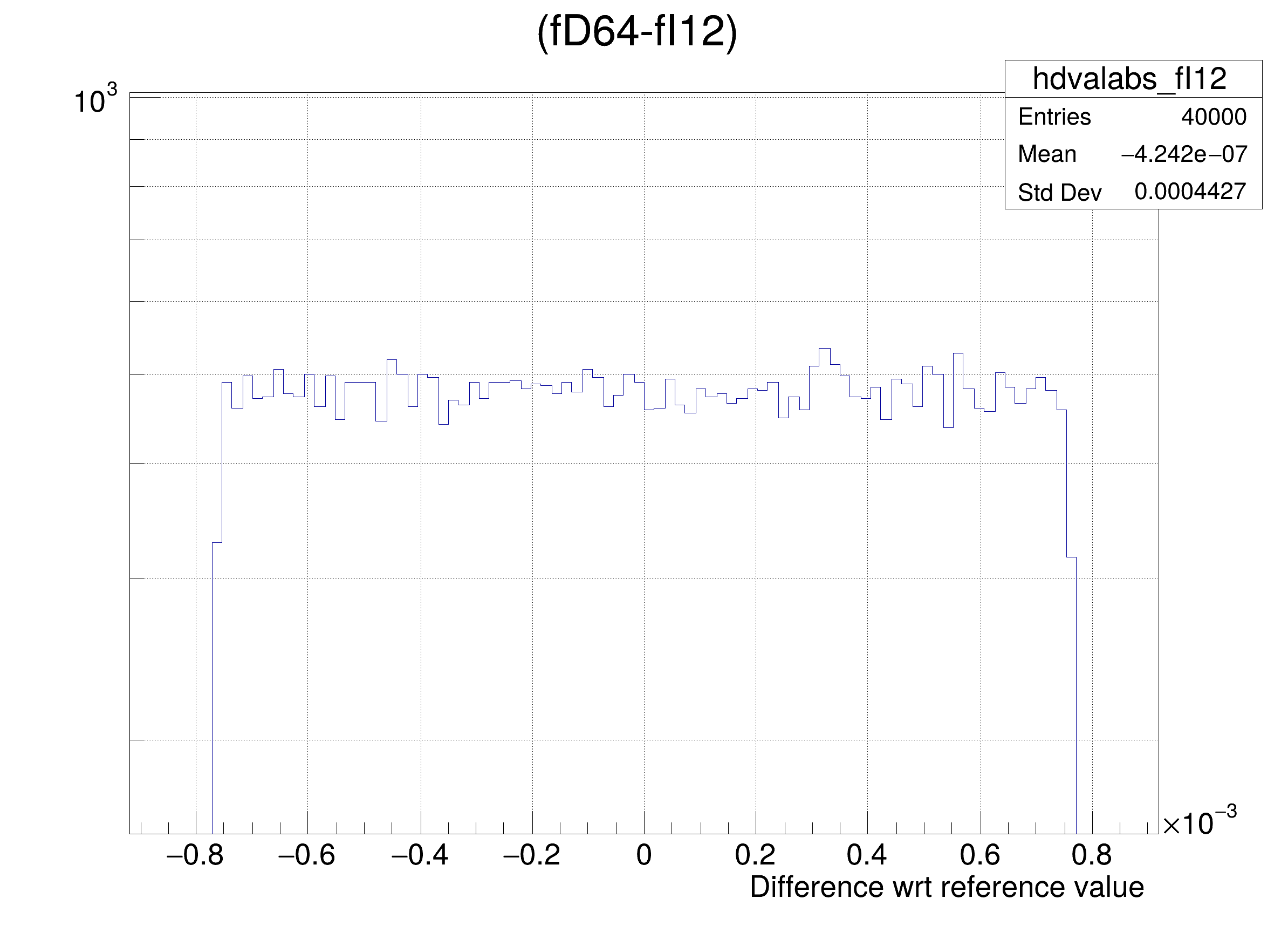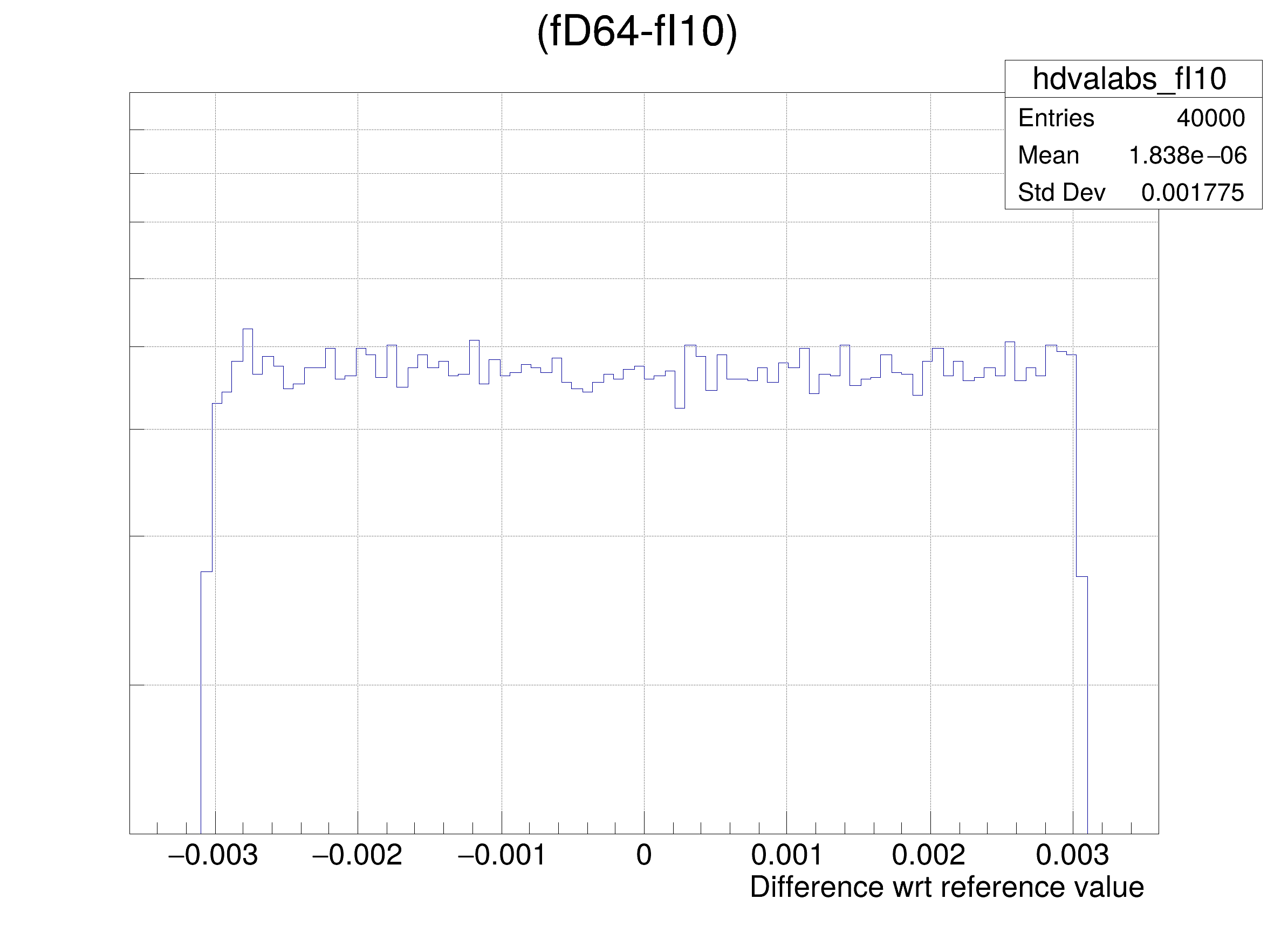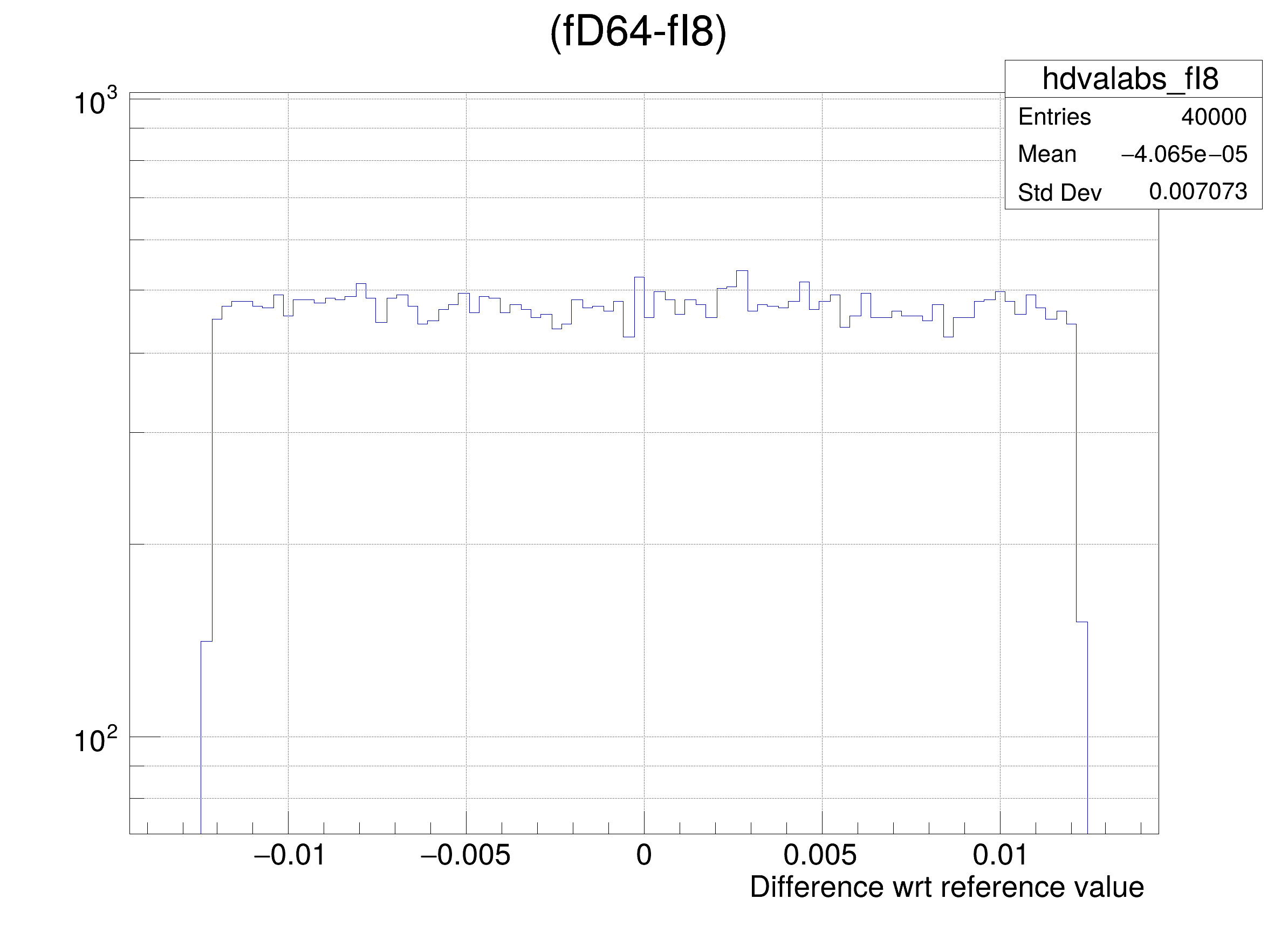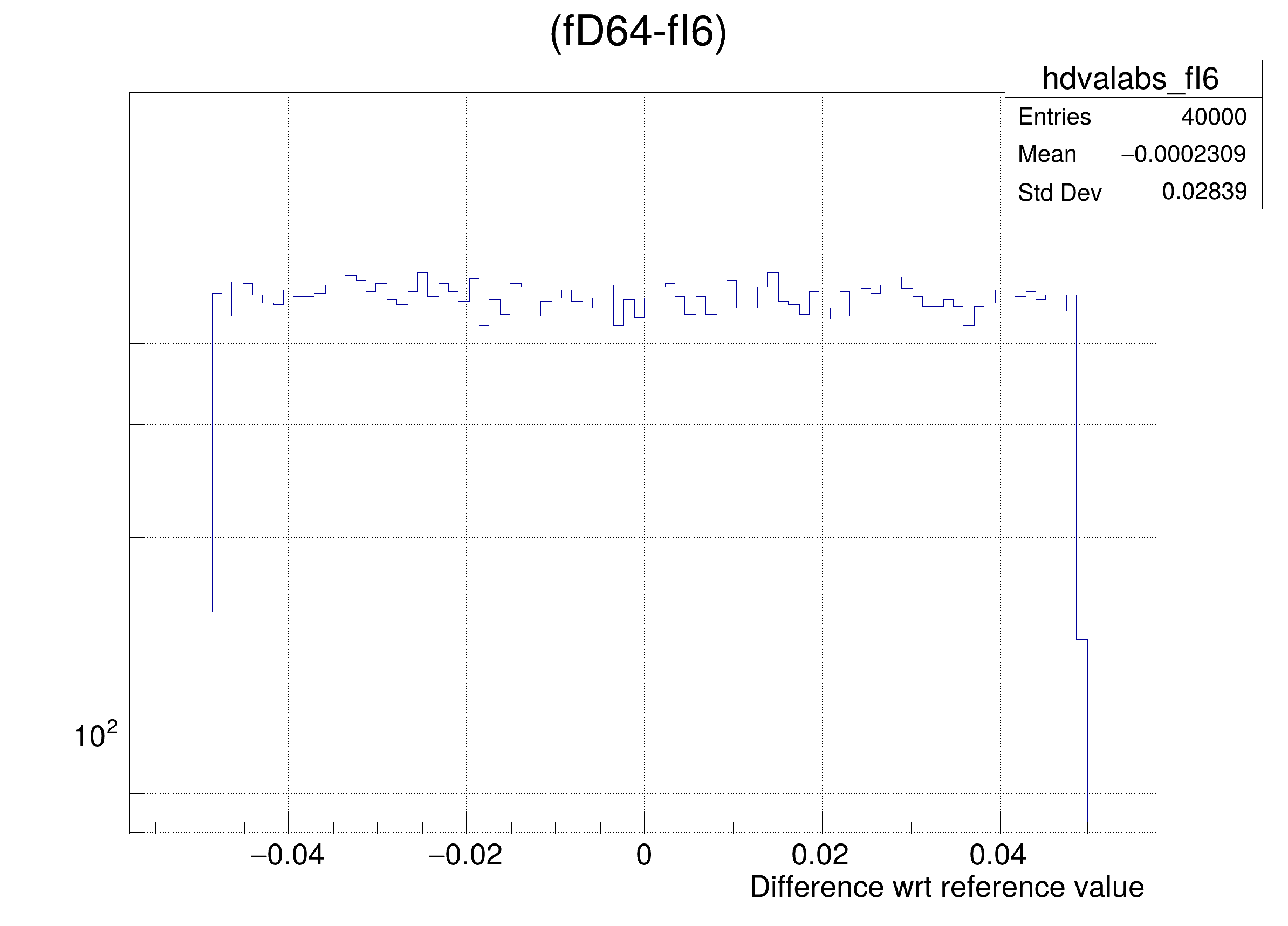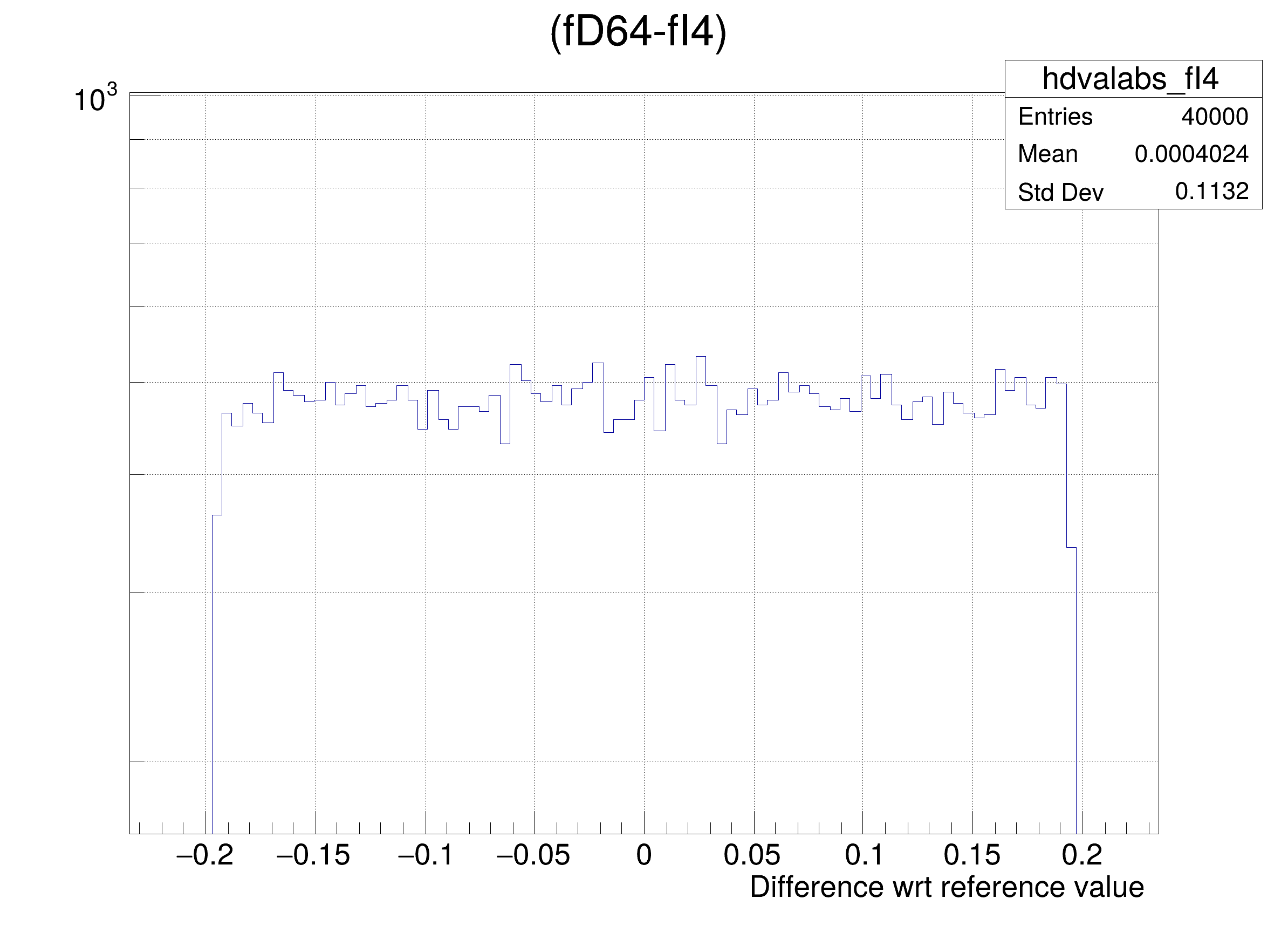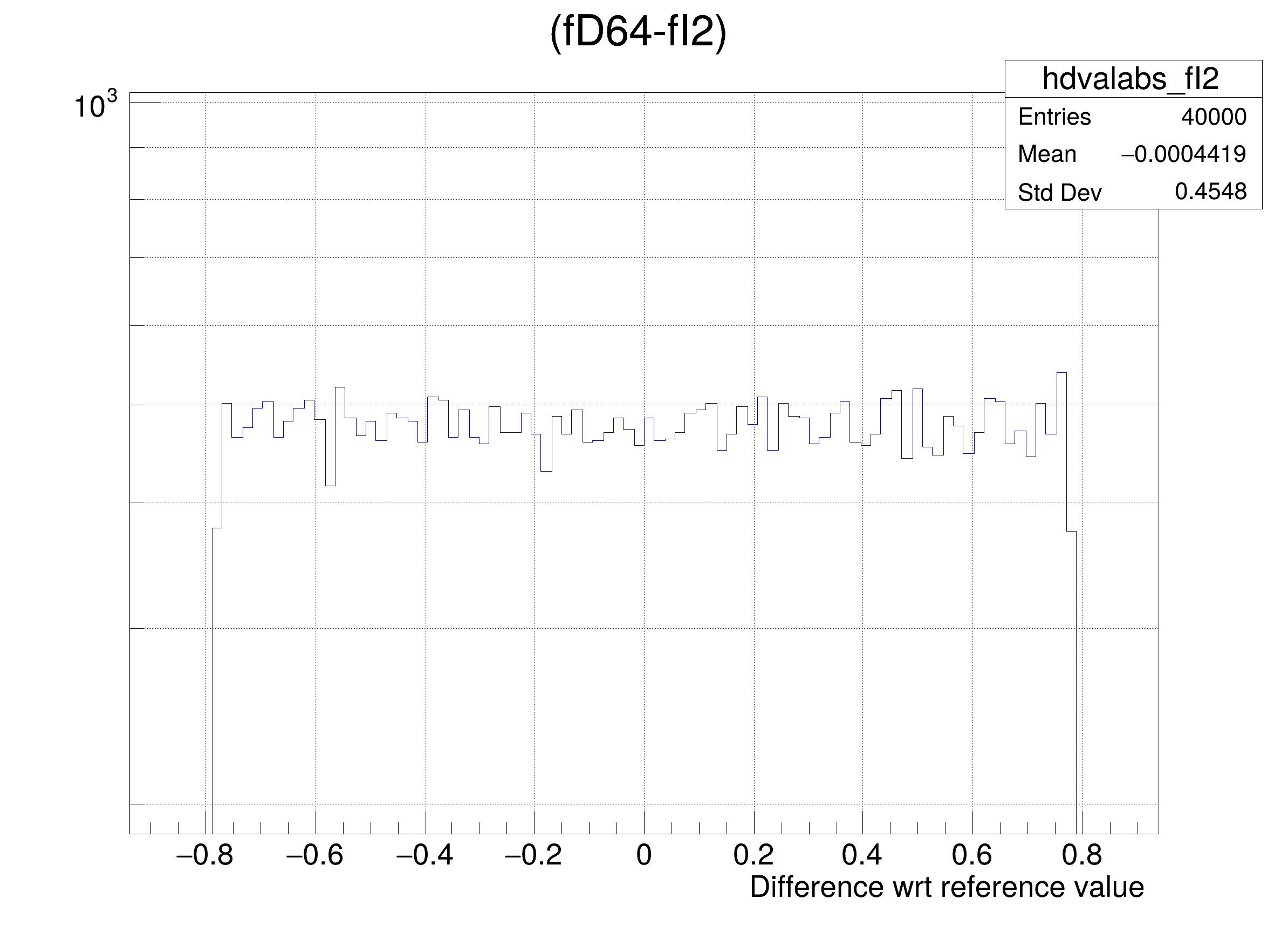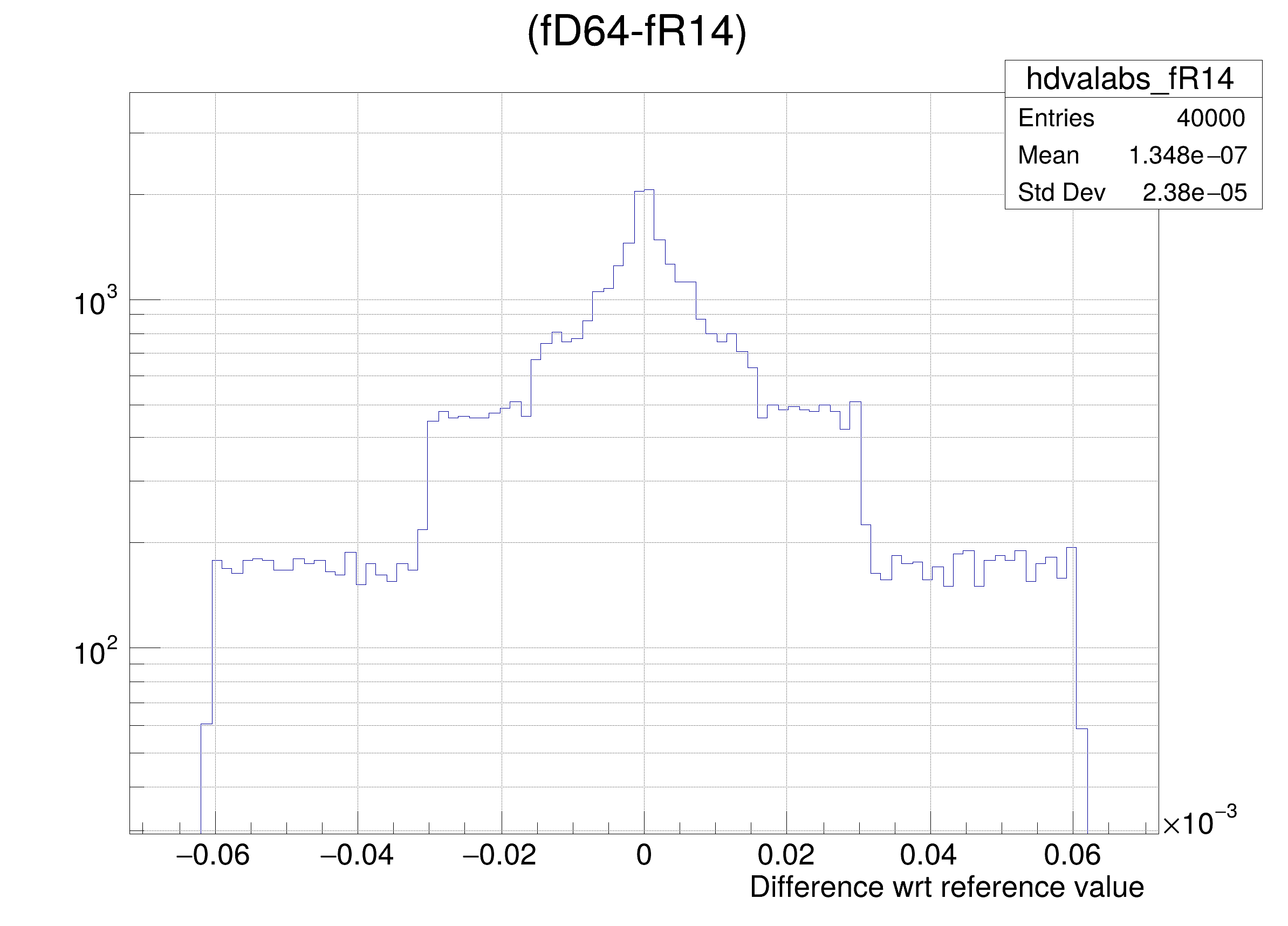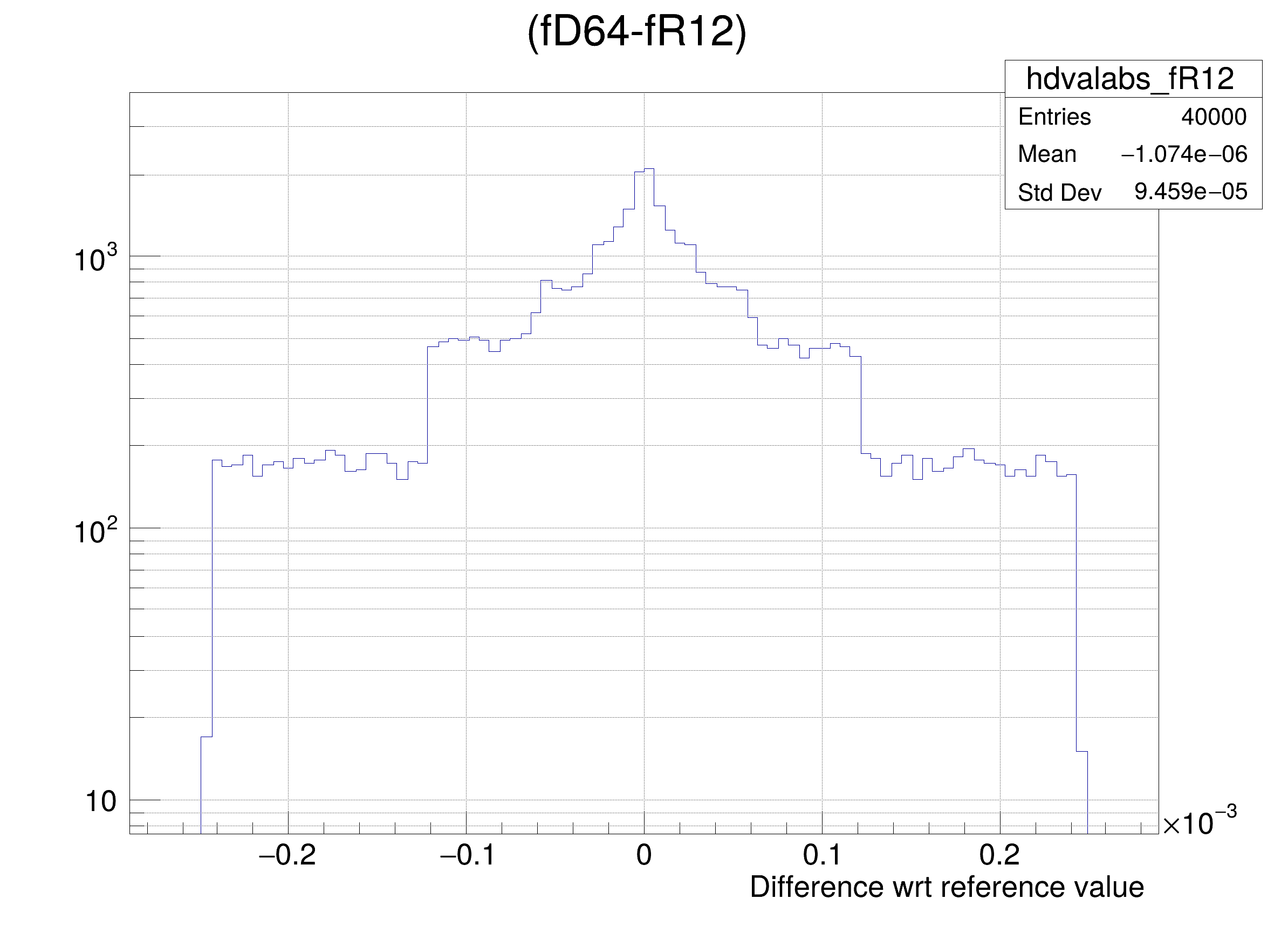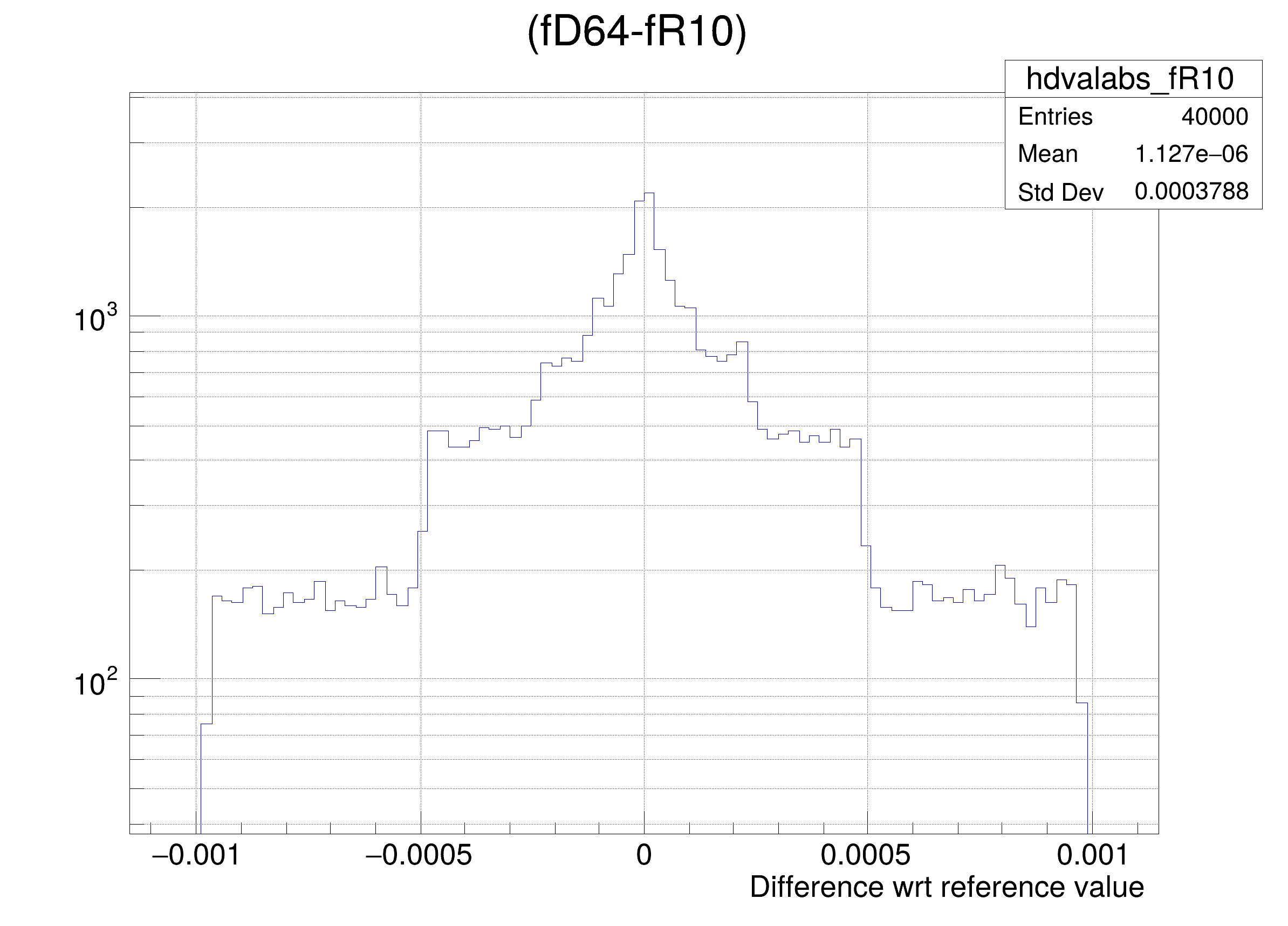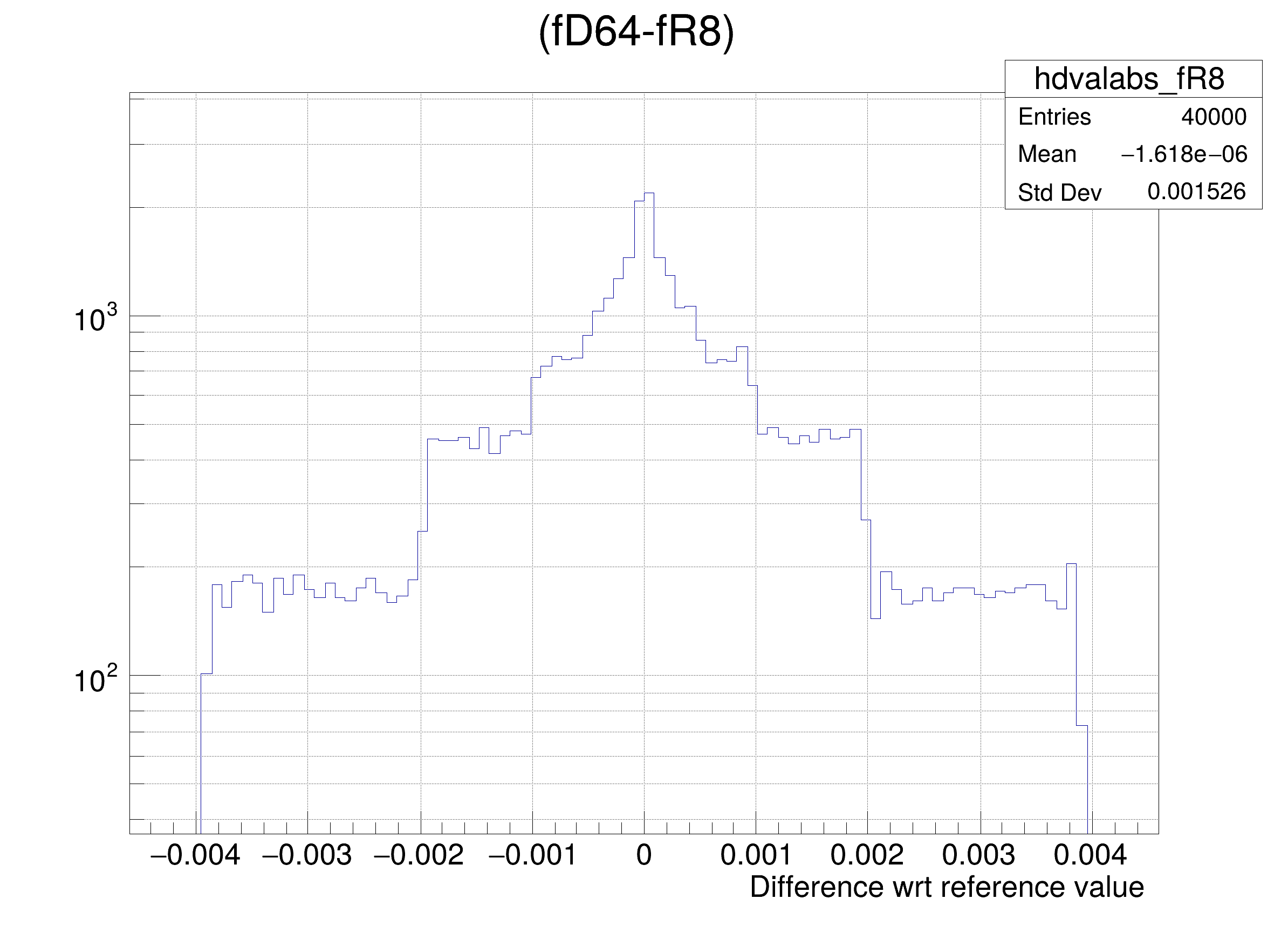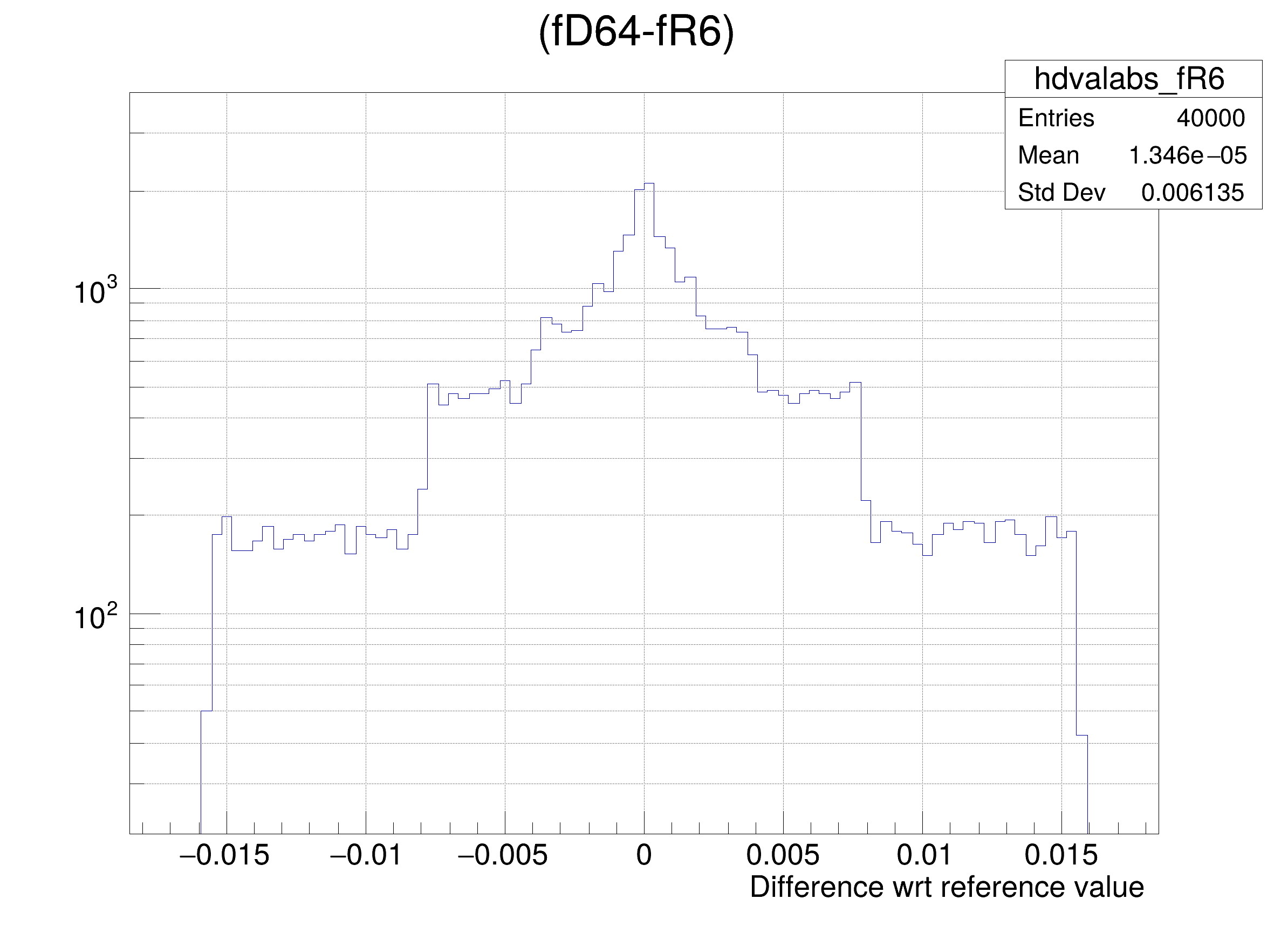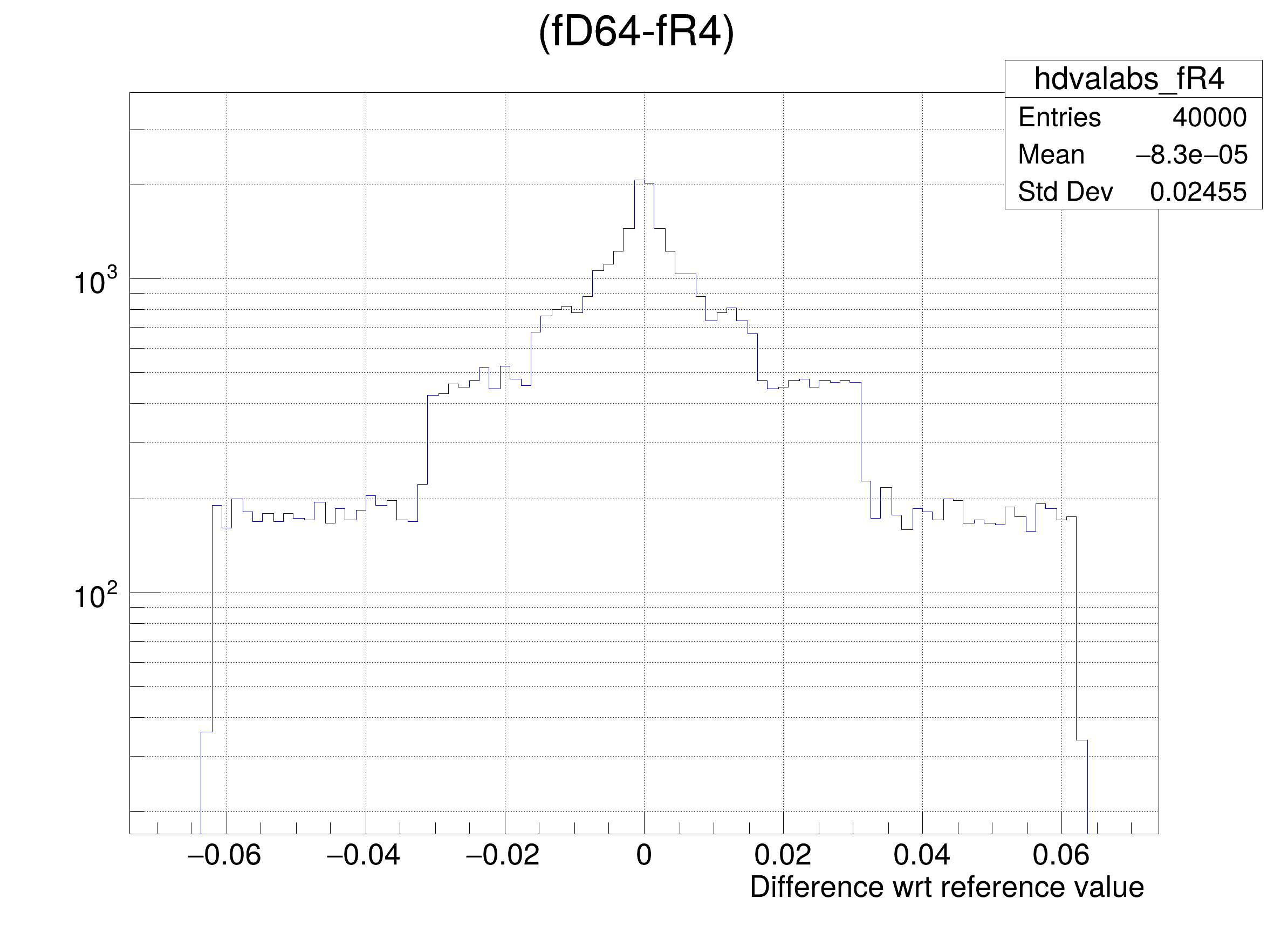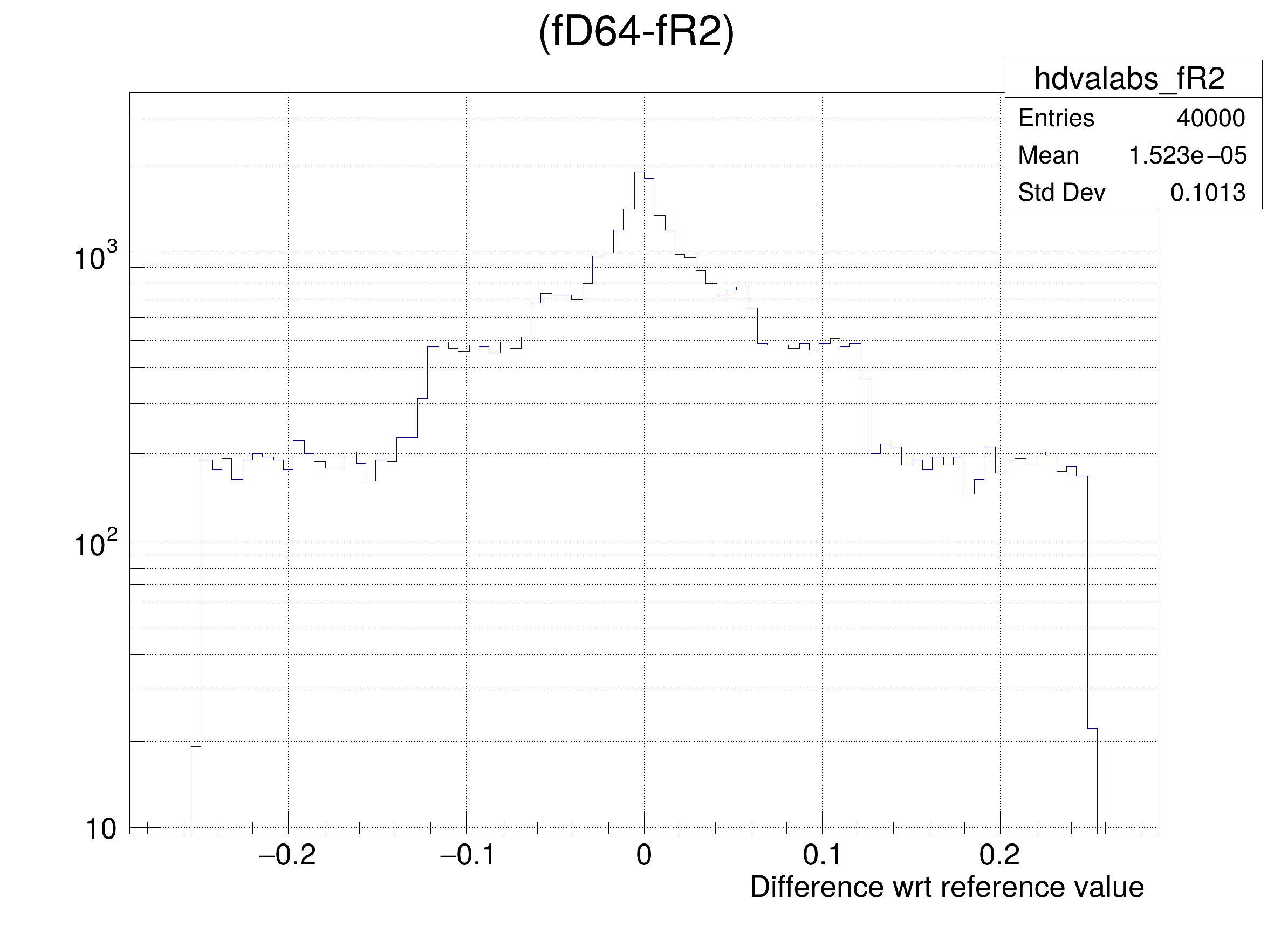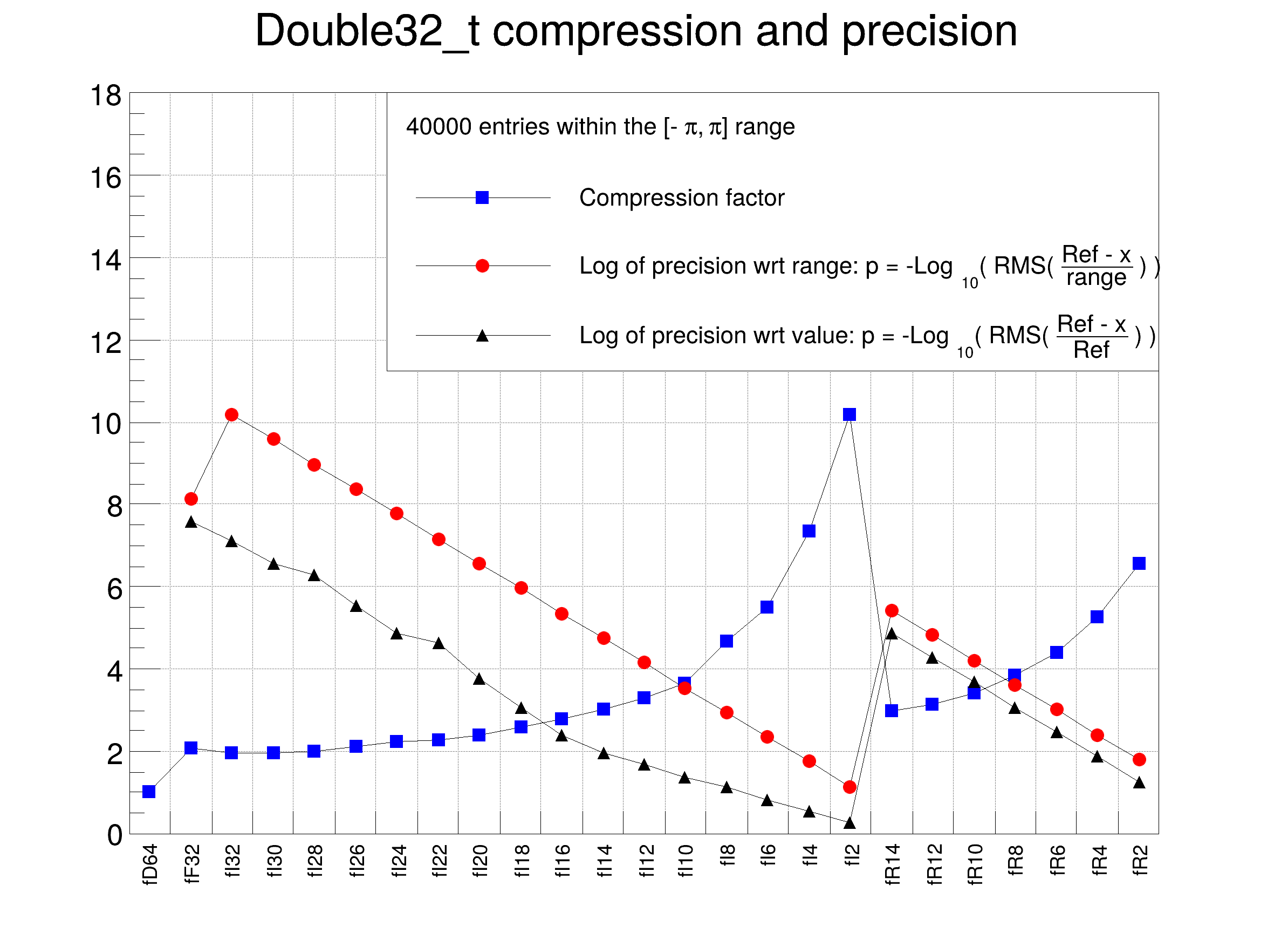#include "ROOT/TSeq.hxx"
#include "TCanvas.h"
#include "TFile.h"
#include "TGraph.h"
#include "TH1.h"
#include "TLegend.h"
#include "TMath.h"
#include "TRandom3.h"
#include "TTree.h"
class DemoDouble32 {
private:
Double_t fD64; // reference member with full double precision
Double32_t fF32; // saved as a 32 bit Float_t
Double32_t fI32; //[-pi,pi] saved as a 32 bit unsigned int
Double32_t fI30; //[-pi,pi,30] saved as a 30 bit unsigned int
Double32_t fI28; //[-pi,pi,28] saved as a 28 bit unsigned int
Double32_t fI26; //[-pi,pi,26] saved as a 26 bit unsigned int
Double32_t fI24; //[-pi,pi,24] saved as a 24 bit unsigned int
Double32_t fI22; //[-pi,pi,22] saved as a 22 bit unsigned int
Double32_t fI20; //[-pi,pi,20] saved as a 20 bit unsigned int
Double32_t fI18; //[-pi,pi,18] saved as a 18 bit unsigned int
Double32_t fI16; //[-pi,pi,16] saved as a 16 bit unsigned int
Double32_t fI14; //[-pi,pi,14] saved as a 14 bit unsigned int
Double32_t fI12; //[-pi,pi,12] saved as a 12 bit unsigned int
Double32_t fI10; //[-pi,pi,10] saved as a 10 bit unsigned int
Double32_t fI8; //[-pi,pi, 8] saved as a 8 bit unsigned int
Double32_t fI6; //[-pi,pi, 6] saved as a 6 bit unsigned int
Double32_t fI4; //[-pi,pi, 4] saved as a 4 bit unsigned int
Double32_t fI2; //[-pi,pi, 2] saved as a 2 bit unsigned int
Double32_t fR14; //[0, 0, 14] saved as a 32 bit float with a 14 bits mantissa
Double32_t fR12; //[0, 0, 12] saved as a 32 bit float with a 12 bits mantissa
Double32_t fR10; //[0, 0, 10] saved as a 32 bit float with a 10 bits mantissa
Double32_t fR8; //[0, 0, 8] saved as a 32 bit float with a 8 bits mantissa
Double32_t fR6; //[0, 0, 6] saved as a 32 bit float with a 6 bits mantissa
Double32_t fR4; //[0, 0, 4] saved as a 32 bit float with a 4 bits mantissa
Double32_t fR2; //[0, 0, 2] saved as a 32 bit float with a 2 bits mantissa
public:
DemoDouble32() = default;
void Set(Double_t ref)
{
fD64 = fF32 = fI32 = fI30 = fI28 = fI26 = fI24 = fI22 = fI20 = fI18 = fI16 = fI14 = fI12 = fI10 = fI8 = fI6 =
fI4 = fI2 = fR14 = fR12 = fR10 = fR8 = fR6 = fR4 = fR2 = ref;
}
};
void double32()
{
const auto nEntries = 40000;
const auto xmax = TMath::Pi();
const auto xmin = -xmax;
// create a Tree with nEntries objects DemoDouble32
TFile::Open("DemoDouble32.root", "recreate");
TTree tree("tree", "DemoDouble32");
DemoDouble32 demoInstance;
auto demoInstanceBranch = tree.Branch("d", "DemoDouble32", &demoInstance, 4000);
for (auto i : ROOT::TSeqI(nEntries)) {
demoInstance.Set(r.Uniform(xmin, xmax));
tree.Fill();
}
tree.Write();
// Now we can proceed with the analysis of the sizes on disk of all branches
// Create the frame histogram and the graphs
auto branches = demoInstanceBranch->GetListOfBranches();
const auto nb = branches->GetEntries();
auto br = static_cast<TBranch *>(branches->At(0));
const Long64_t zip64 = br->GetZipBytes();
auto h = new TH1F("h", "Double32_t compression and precision", nb, 0, nb);
h->SetMaximum(18);
h->SetStats(0);
auto gcx = new TGraph();
gcx->SetName("gcx");
gcx->SetMarkerStyle(kFullSquare);
gcx->SetMarkerColor(kBlue);
auto gdrange = new TGraph();
gdrange->SetName("gdrange");
gdrange->SetMarkerStyle(kFullCircle);
gdrange->SetMarkerColor(kRed);
auto gdval = new TGraph();
gdval->SetName("gdval");
gdval->SetMarkerStyle(kFullTriangleUp);
gdval->SetMarkerColor(kBlack);
// loop on branches to get the precision and compression factors
for (auto i : ROOT::TSeqI(nb)) {
auto br = static_cast<TBranch *>(branches->At(i));
const auto brName = br->GetName();
h->GetXaxis()->SetBinLabel(i + 1, brName);
const auto cx = double(zip64) / br->GetZipBytes();
gcx->SetPoint(i, i + 0.5, cx);
if (i == 0 ) continue;
tree.Draw(Form("(fD64-%s)/(%g)", brName, xmax - xmin), "", "goff");
const auto rmsDrange = TMath::RMS(nEntries, tree.GetV1());
const auto drange = TMath::Max(0., -TMath::Log10(rmsDrange));
gdrange->SetPoint(i-1, i + 0.5, drange);
tree.Draw(Form("(fD64-%s)/fD64", brName), "", "goff");
const auto rmsDVal = TMath::RMS(nEntries, tree.GetV1());
const auto dval = TMath::Max(0., -TMath::Log10(rmsDVal));
gdval->SetPoint(i-1, i + 0.5, dval);
tree.Draw(Form("(fD64-%s) >> hdvalabs_%s", brName, brName), "", "goff");
auto hdval = gDirectory->Get<TH1F>(Form("hdvalabs_%s", brName));
hdval->GetXaxis()->SetTitle("Difference wrt reference value");
auto c = new TCanvas(brName, brName, 800, 600);
c->SetGrid();
c->SetLogy();
hdval->DrawClone();
}
auto c1 = new TCanvas("c1", "c1", 800, 600);
c1->SetGrid();
h->Draw();
h->GetXaxis()->LabelsOption("v");
gcx->Draw("lp");
gdrange->Draw("lp");
gdval->Draw("lp");
// Finally build a legend
auto legend = new TLegend(0.3, 0.6, 0.9, 0.9);
legend->SetHeader(Form("%d entries within the [-#pi, #pi] range", nEntries));
legend->AddEntry(gdrange, "Log of precision wrt range: p = -Log_{10}( RMS( #frac{Ref - x}{range} ) ) ", "lp");
legend->AddEntry(gdval, "Log of precision wrt value: p = -Log_{10}( RMS( #frac{Ref - x}{Ref} ) ) ", "lp");
legend->Draw();
}

Definition in file double32.C.

c
#define c(i)
Definition: RSha256.hxx:101
TBranch::GetZipBytes
Long64_t GetZipBytes(Option_t *option="") const
Return total number of zip bytes in the branch if option ="*" includes all sub-branches of this branc...
Definition: TBranch.cxx:2131
TMath::Max
Short_t Max(Short_t a, Short_t b)
Definition: TMathBase.h:212
tree
Definition: tree.py:1
Form
char * Form(const char *fmt,...)
r
ROOT::R::TRInterface & r
Definition: Object.C:4
TGraph.h
xmax
float xmax
Definition: THbookFile.cxx:95
Long64_t
long long Long64_t
Definition: RtypesCore.h:73
TLegend.h
TTree
A TTree represents a columnar dataset.
Definition: TTree.h:79
TFile::Open
static TFile * Open(const char *name, Option_t *option="", const char *ftitle="", Int_t compress=ROOT::RCompressionSetting::EDefaults::kUseCompiledDefault, Int_t netopt=0)
Create / open a file.
Definition: TFile.cxx:3995
Sets the header, which is the "title" that appears at the top of the legend.
Definition: TLegend.cxx:1099
TCanvas.h
TTree.h
TFile.h
TBranch
A TTree is a list of TBranches.
Definition: TBranch.h:89
kBlack
@ kBlack
Definition: Rtypes.h:65
TMath::Pi
constexpr Double_t Pi()
Definition: TMath.h:37
kFullCircle
@ kFullCircle
Definition: TAttMarker.h:51
TMath::Log10
Double_t Log10(Double_t x)
Definition: TMath.h:764
kFullTriangleUp
@ kFullTriangleUp
Definition: TAttMarker.h:51
xmin
float xmin
Definition: THbookFile.cxx:95
TRandom3
Random number generator class based on M.
Definition: TRandom3.h:27
h
#define h(i)
Definition: RSha256.hxx:106
TSeq.hxx
Double32_t
double Double32_t
Definition: RtypesCore.h:60
TLegendEntry * AddEntry(const TObject *obj, const char *label="", Option_t *option="lpf")
Add a new entry to this legend.
Definition: TLegend.cxx:330
gDirectory
#define gDirectory
Definition: TDirectory.h:236
TRandom3.h
double
double
Definition: Converters.cxx:921
TMath::RMS
Double_t RMS(Long64_t n, const T *a, const Double_t *w=0)
Return the Standard Deviation of an array a with length n.
Definition: TMath.h:1167
kRed
@ kRed
Definition: Rtypes.h:66
TNamed::SetTitle
virtual void SetTitle(const char *title="")
Set the title of the TNamed.
Definition: TNamed.cxx:164
kFullSquare
@ kFullSquare
Definition: TAttMarker.h:51
Double_t
double Double_t
Definition: RtypesCore.h:59
TGraph
A TGraph is an object made of two arrays X and Y with npoints each.
Definition: TGraph.h:41
TCanvas
The Canvas class.
Definition: TCanvas.h:23
TH1F
1-D histogram with a float per channel (see TH1 documentation)}
Definition: TH1.h:575
kBlue
@ kBlue
Definition: Rtypes.h:66
TLegend::Draw
virtual void Draw(Option_t *option="")
Draw this legend with its current attributes.
Definition: TLegend.cxx:423
TLegend
This class displays a legend box (TPaveText) containing several legend entries.
Definition: TLegend.h:23
ROOT::TSeq
A pseudo container class which is a generator of indices.
Definition: TSeq.hxx:66
TNamed::GetName
virtual const char * GetName() const
Returns name of object.
Definition: TNamed.h:47
TH1::GetXaxis
TAxis * GetXaxis()